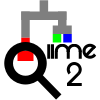# Selecting features for DEICODE biplot (Emperor vs R)

Hellocommunity,

While making a biplot from Deicode results in R basing on this tuto (How to make pcoa biplot in R using q2-deicode ordination), I noticed that different features where being selected to plot as arrows in the graph in R and Emperor.

In the R code, features to plot are selected with the following cmd, `mutate(a=sqrt(PC1^2+PC2^2)) %>% top_n(8, a)` according to the tuto; after some attempts I added the PC3 in the cmd like `mutate(a=sqrt(PC1^2+PC2^2+PC3^2)) %>% top_n(8, a)` and the selected features between Emperor and R were the same.

Questions: is it correct to add the PC3 in the R calculation even if that "dimension" isn't going to be plotted? Which way is the best to select the features?.
Also, in the Emperor plot there is a Confidence value related to the arrows, I haven't been able to find it in the ordination file, where does it come from? should be taken into account while plotting in R?.

Cheers,
Luis Alfonso.

2 Likes

`mutate(a=sqrt(PC1^2+PC2^2)) %>% top_n(8, a)` is Pythagorean's theorem solving for the hypotenuse (i.e. distance from the origin) and then taking the 8 features that have the largest effect on PC1 and PC2. Perhaps the distance of PC3 is negligible and thus didn't change your value for `a`.

Not sure about the confidence value and how it is calculated; hopefully someone else could help you out there.

1 Like